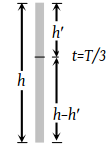A ball is released from the top of a tower of height h meters. It takes T seconds to reach the ground. What is the position of the ball in T/3 seconds

(1) h/9 meters from the ground

(2) 7h/9 meters from the ground

(3) 8h/9 meters from the ground

(4) 17h/18 meters from the ground

Concept Videos :-

#13-Graphical-Meaning-of-Instantaneous
#14-Solved-Examples-7
#12-Instantaneous-Velocity

Concept Questions :-

Instantaneous speed and instantaneous velocity

(3) $\because \text{\hspace{0.17em}\hspace{0.17em}}h=ut+\frac{1}{2}g{t}^{2}⇒h=\frac{1}{2}g{T}^{2}$After $\frac{T}{3}$ seconds, the position of ball,

${h}^{\text{'}}=0+\frac{1}{2}g{\left(\frac{T}{3}\right)}^{2}=\frac{1}{2}×\frac{g}{9}×{T}^{2}$

$h\text{'}=\frac{1}{2}×\frac{g}{9}×{T}^{2}=\frac{h}{9}m$ from top

∴ Position of ball from ground $=h-\frac{h}{9}=\frac{8\text{\hspace{0.17em}}h}{9}m.$

Difficulty Level:

• 16%
• 18%
• 63%
• 5%